# Sourcepredict example2: Estimating source proportions¶

For this example, we’ll reuse the dog, human, and soil dataset.
But unlike example1, here we will mix samples from different sources and estimate the mixing proportions with Sourcepredict and Sourcetracker2

## Preparing mixed samples¶

:

import pandas as pd
from plotnine import *
import numpy as np

:

cnt = pd.read_csv("../data/modern_gut_microbiomes_sources.csv", index_col=0)


As in example 1, we’ll first split the dataset into training (95%) and testing(5%)

:

cnt_train = cnt.sample(frac=0.95, axis=1, random_state=42)
cnt_test = cnt.drop(cnt_train.columns, axis=1)
train_labels = labels.loc[cnt_train.columns,:]
test_labels = labels.loc[cnt_test.columns,:]

:

test_labels['labels'].value_counts()

:

Homo_sapiens        13
Canis_familiaris     8
Soil                 1
Name: labels, dtype: int64

:

cnt_test.head()

:

SRR059440 SRR1930140 SRR1761708 SRR1761664 SRR1761667 SRR1761674 SRR7658684 SRR7658622 SRR7658689 SRR7658619 ... SRR5898940 ERR1914224 ERR1915611 ERR1915293 ERR1914207 ERR1915420 ERR1916218 ERR1913675 ERR1914667 SRR3578645
TAXID
0 19805534.0 7267728.0 18530434.0 2460493.0 3324349.0 2835521.0 5565044.0 18783402.0 6319253.0 18641694.0 ... 1658949.0 3442424.0 1529589.0 1765224.0 1815426.0 1364019.0 1558043.0 1617964.0 1557538.0 736.0
6 0.0 0.0 85.0 0.0 0.0 0.0 0.0 542.0 0.0 217.0 ... 0.0 0.0 0.0 0.0 0.0 0.0 0.0 0.0 0.0 0.0
7 0.0 0.0 85.0 0.0 0.0 0.0 0.0 542.0 0.0 217.0 ... 0.0 0.0 0.0 0.0 0.0 0.0 0.0 0.0 0.0 0.0
9 239.0 88.0 115.0 55.0 189.0 91.0 72.0 100.0 152.0 209.0 ... 0.0 51.0 69.0 60.0 56.0 106.0 51.0 69.0 62.0 0.0
10 163.0 177.0 112.0 76.0 164.0 109.0 220.0 84.0 180.0 175.0 ... 0.0 202.0 80.0 67.0 73.0 0.0 80.0 77.0 110.0 0.0

5 rows × 22 columns

We then create a function to randomly select a sample from each source (dog as $$s_{dog}$$ and human as $$s_{human}$$), and combine such as the new sample $$s_{mixed} = p1*s_{dog} + p1*s_{human}$$

:

def create_mixed_sample(cnt, labels, p1, samp_name, seed):
rand_dog = labels.query('labels == "Canis_familiaris"').sample(1, random_state = seed).index
rand_human = labels.query('labels == "Homo_sapiens"').sample(1, random_state = seed).index
dog_samp = cnt[rand_dog]*p1
human_samp = cnt[rand_human]*(1-p1)
comb = dog_samp + human_samp
comb = comb.rename(samp_name)
meta = pd.DataFrame({'human_sample':[rand_human],'dog_sample':[rand_dog], 'human_prop':[(1-p1)], 'dog_prop':[p1]}, index=[samp_name])
return(comb, meta)


We run this function for a range of mixed proportions (0 to 100%, by 10%), 3 time for each mix

:

mixed_samp = []
mixed_meta = []
nb = 1
for i in range(3):
for p1 in np.arange(0,1.1,0.1):
s = create_mixed_sample(cnt=cnt_test, labels=test_labels, p1=p1, samp_name=f"mixed_sample_{nb}", seed = int(100*p1))
mixed_samp.append(s)
mixed_meta.append(s)
nb += 1

:

mixed_samples = pd.concat(mixed_samp, axis=1, keys=[s.name for s in mixed_samp]).astype(int)

:

mixed_sample_1 mixed_sample_2 mixed_sample_3 mixed_sample_4 mixed_sample_5 mixed_sample_6 mixed_sample_7 mixed_sample_8 mixed_sample_9 mixed_sample_10 ... mixed_sample_24 mixed_sample_25 mixed_sample_26 mixed_sample_27 mixed_sample_28 mixed_sample_29 mixed_sample_30 mixed_sample_31 mixed_sample_32 mixed_sample_33
TAXID
0 5565044 2390966 15338330 14408501 7983985 5100662 8740624 3251030 3418809 2103402 ... 2390966 15338330 14408501 7983985 5100662 8740624 3251030 3418809 2103402 1529589
6 0 0 433 0 66 26 0 0 0 0 ... 0 433 0 66 26 0 0 0 0 0
7 0 0 433 0 66 26 0 0 0 0 ... 0 433 0 66 26 0 0 0 0 0
9 72 55 90 184 98 141 159 74 78 70 ... 55 90 184 98 141 159 74 78 70 69
10 220 75 83 136 276 117 65 109 194 89 ... 75 83 136 276 117 65 109 194 89 80

5 rows × 33 columns

:

mixed_metadata = pd.concat(mixed_meta)

:

human_sample dog_sample human_prop dog_prop
mixed_sample_1 SRR7658684 ERR1913675 1.0 0.0
mixed_sample_2 SRR1761664 ERR1915293 0.9 0.1
mixed_sample_3 SRR7658622 ERR1916218 0.8 0.2
mixed_sample_4 SRR059440 ERR1914207 0.7 0.3
mixed_sample_5 SRR7658624 ERR1914667 0.6 0.4
mixed_sample_6 SRR7658608 ERR1915420 0.5 0.5
mixed_sample_7 SRR059440 ERR1915420 0.4 0.6
mixed_sample_8 SRR1930140 ERR1915611 0.3 0.7
mixed_sample_9 SRR1761667 ERR1914224 0.2 0.8
mixed_sample_10 SRR1930140 ERR1915611 0.1 0.9
mixed_sample_11 SRR7658624 ERR1915611 0.0 1.0
mixed_sample_12 SRR7658684 ERR1913675 1.0 0.0
mixed_sample_13 SRR1761664 ERR1915293 0.9 0.1
mixed_sample_14 SRR7658622 ERR1916218 0.8 0.2
mixed_sample_15 SRR059440 ERR1914207 0.7 0.3
mixed_sample_16 SRR7658624 ERR1914667 0.6 0.4
mixed_sample_17 SRR7658608 ERR1915420 0.5 0.5
mixed_sample_18 SRR059440 ERR1915420 0.4 0.6
mixed_sample_19 SRR1930140 ERR1915611 0.3 0.7
mixed_sample_20 SRR1761667 ERR1914224 0.2 0.8
mixed_sample_21 SRR1930140 ERR1915611 0.1 0.9
mixed_sample_22 SRR7658624 ERR1915611 0.0 1.0
mixed_sample_23 SRR7658684 ERR1913675 1.0 0.0
mixed_sample_24 SRR1761664 ERR1915293 0.9 0.1
mixed_sample_25 SRR7658622 ERR1916218 0.8 0.2
mixed_sample_26 SRR059440 ERR1914207 0.7 0.3
mixed_sample_27 SRR7658624 ERR1914667 0.6 0.4
mixed_sample_28 SRR7658608 ERR1915420 0.5 0.5
mixed_sample_29 SRR059440 ERR1915420 0.4 0.6
mixed_sample_30 SRR1930140 ERR1915611 0.3 0.7
mixed_sample_31 SRR1761667 ERR1914224 0.2 0.8
mixed_sample_32 SRR1930140 ERR1915611 0.1 0.9
mixed_sample_33 SRR7658624 ERR1915611 0.0 1.0

Now we can export the new “test” (sink) table to csv for sourcepredict

:

mixed_samples.to_csv('mixed_samples_cnt.csv')


As well as the source count and labels table for the sources

:

train_labels.to_csv('train_labels.csv')
cnt_train.to_csv('sources_cnt.csv')


## Sourcepredict¶

### With KNN machine learning¶

For running Sourcepredict, we’ll change two parameters from their default values: - -me The default method used by Sourcepredict is T-SNE which a non-linear type of embedding, i.e. the distance between points doesn’t reflext their actual distance in the original dimensions, to achieve a better clustering, which is good for source prediction. Because here we’re more interested in source proportion estimation, rather than source prediction, we’ll choose a Multi Dimensional Scaling (MDS) which is a type of linear embedding, where the distance between points in the lower dimension match more the distances in the embedding in lower dimension, which is better for source proportion estimation. - -kne which is the number of neighbors in KNN algorithm: we use all neighbors to reflect a more global contribution of samples to the proportion estimation, instead of only the immediate neighbors. This will affect negatively the source prediction, but give better source proportion estimations

:

%%time
!python ../sourcepredict -s sources_cnt.csv \
-l train_labels.csv \
-kne all\
-me mds \
-e mixed_embedding.csv \
-t 6 \
mixed_samples_cnt.csv

/Users/borry/miniconda3/envs/sourcepredict/lib/python3.7/site-packages/sklearn/externals/joblib/__init__.py:15: DeprecationWarning: sklearn.externals.joblib is deprecated in 0.21 and will be removed in 0.23. Please import this functionality directly from joblib, which can be installed with: pip install joblib. If this warning is raised when loading pickled models, you may need to re-serialize those models with scikit-learn 0.21+.
warnings.warn(msg, category=DeprecationWarning)
Step 1: Checking for unknown proportion
== Sample: mixed_sample_1 ==
Normalizing (GMPR)
Computing Bray-Curtis distance
Performing MDS embedding in 2 dimensions
KNN machine learning
Training KNN classifier on 6 cores...
-> Testing Accuracy: 1.0
----------------------
- Sample: mixed_sample_1
known:98.48%
unknown:1.52%
== Sample: mixed_sample_2 ==
Normalizing (GMPR)
Computing Bray-Curtis distance
Performing MDS embedding in 2 dimensions
KNN machine learning
Training KNN classifier on 6 cores...
-> Testing Accuracy: 1.0
----------------------
- Sample: mixed_sample_2
known:98.48%
unknown:1.52%
== Sample: mixed_sample_3 ==
Normalizing (GMPR)
Computing Bray-Curtis distance
Performing MDS embedding in 2 dimensions
KNN machine learning
Training KNN classifier on 6 cores...
-> Testing Accuracy: 0.98
----------------------
- Sample: mixed_sample_3
known:98.49%
unknown:1.51%
== Sample: mixed_sample_4 ==
Normalizing (GMPR)
Computing Bray-Curtis distance
Performing MDS embedding in 2 dimensions
KNN machine learning
Training KNN classifier on 6 cores...
-> Testing Accuracy: 1.0
----------------------
- Sample: mixed_sample_4
known:98.49%
unknown:1.51%
== Sample: mixed_sample_5 ==
Normalizing (GMPR)
Computing Bray-Curtis distance
Performing MDS embedding in 2 dimensions
KNN machine learning
Training KNN classifier on 6 cores...
-> Testing Accuracy: 1.0
----------------------
- Sample: mixed_sample_5
known:98.48%
unknown:1.52%
== Sample: mixed_sample_6 ==
Normalizing (GMPR)
Computing Bray-Curtis distance
Performing MDS embedding in 2 dimensions
KNN machine learning
Training KNN classifier on 6 cores...
-> Testing Accuracy: 1.0
----------------------
- Sample: mixed_sample_6
known:98.48%
unknown:1.52%
== Sample: mixed_sample_7 ==
Normalizing (GMPR)
Computing Bray-Curtis distance
Performing MDS embedding in 2 dimensions
KNN machine learning
Training KNN classifier on 6 cores...
-> Testing Accuracy: 1.0
----------------------
- Sample: mixed_sample_7
known:98.48%
unknown:1.52%
== Sample: mixed_sample_8 ==
Normalizing (GMPR)
Computing Bray-Curtis distance
Performing MDS embedding in 2 dimensions
KNN machine learning
Training KNN classifier on 6 cores...
-> Testing Accuracy: 1.0
----------------------
- Sample: mixed_sample_8
known:98.48%
unknown:1.52%
== Sample: mixed_sample_9 ==
Normalizing (GMPR)
Computing Bray-Curtis distance
Performing MDS embedding in 2 dimensions
KNN machine learning
Training KNN classifier on 6 cores...
-> Testing Accuracy: 1.0
----------------------
- Sample: mixed_sample_9
known:98.48%
unknown:1.52%
== Sample: mixed_sample_10 ==
Normalizing (GMPR)
Computing Bray-Curtis distance
Performing MDS embedding in 2 dimensions
KNN machine learning
Training KNN classifier on 6 cores...
-> Testing Accuracy: 1.0
----------------------
- Sample: mixed_sample_10
known:98.48%
unknown:1.52%
== Sample: mixed_sample_11 ==
Normalizing (GMPR)
Computing Bray-Curtis distance
Performing MDS embedding in 2 dimensions
KNN machine learning
Training KNN classifier on 6 cores...
-> Testing Accuracy: 1.0
----------------------
- Sample: mixed_sample_11
known:98.48%
unknown:1.52%
== Sample: mixed_sample_12 ==
Normalizing (GMPR)
Computing Bray-Curtis distance
Performing MDS embedding in 2 dimensions
KNN machine learning
Training KNN classifier on 6 cores...
-> Testing Accuracy: 1.0
----------------------
- Sample: mixed_sample_12
known:98.48%
unknown:1.52%
== Sample: mixed_sample_13 ==
Normalizing (GMPR)
Computing Bray-Curtis distance
Performing MDS embedding in 2 dimensions
KNN machine learning
Training KNN classifier on 6 cores...
-> Testing Accuracy: 1.0
----------------------
- Sample: mixed_sample_13
known:98.48%
unknown:1.52%
== Sample: mixed_sample_14 ==
Normalizing (GMPR)
Computing Bray-Curtis distance
Performing MDS embedding in 2 dimensions
KNN machine learning
Training KNN classifier on 6 cores...
-> Testing Accuracy: 0.98
----------------------
- Sample: mixed_sample_14
known:98.49%
unknown:1.51%
== Sample: mixed_sample_15 ==
Normalizing (GMPR)
Computing Bray-Curtis distance
Performing MDS embedding in 2 dimensions
KNN machine learning
Training KNN classifier on 6 cores...
-> Testing Accuracy: 1.0
----------------------
- Sample: mixed_sample_15
known:98.49%
unknown:1.51%
== Sample: mixed_sample_16 ==
Normalizing (GMPR)
Computing Bray-Curtis distance
Performing MDS embedding in 2 dimensions
KNN machine learning
Training KNN classifier on 6 cores...
-> Testing Accuracy: 1.0
----------------------
- Sample: mixed_sample_16
known:98.48%
unknown:1.52%
== Sample: mixed_sample_17 ==
Normalizing (GMPR)
Computing Bray-Curtis distance
Performing MDS embedding in 2 dimensions
KNN machine learning
Training KNN classifier on 6 cores...
-> Testing Accuracy: 1.0
----------------------
- Sample: mixed_sample_17
known:98.48%
unknown:1.52%
== Sample: mixed_sample_18 ==
Normalizing (GMPR)
Computing Bray-Curtis distance
Performing MDS embedding in 2 dimensions
KNN machine learning
Training KNN classifier on 6 cores...
-> Testing Accuracy: 1.0
----------------------
- Sample: mixed_sample_18
known:98.48%
unknown:1.52%
== Sample: mixed_sample_19 ==
Normalizing (GMPR)
Computing Bray-Curtis distance
Performing MDS embedding in 2 dimensions
KNN machine learning
Training KNN classifier on 6 cores...
-> Testing Accuracy: 1.0
----------------------
- Sample: mixed_sample_19
known:98.48%
unknown:1.52%
== Sample: mixed_sample_20 ==
Normalizing (GMPR)
Computing Bray-Curtis distance
Performing MDS embedding in 2 dimensions
KNN machine learning
Training KNN classifier on 6 cores...
-> Testing Accuracy: 1.0
----------------------
- Sample: mixed_sample_20
known:98.48%
unknown:1.52%
== Sample: mixed_sample_21 ==
Normalizing (GMPR)
Computing Bray-Curtis distance
Performing MDS embedding in 2 dimensions
KNN machine learning
Training KNN classifier on 6 cores...
-> Testing Accuracy: 1.0
----------------------
- Sample: mixed_sample_21
known:98.48%
unknown:1.52%
== Sample: mixed_sample_22 ==
Normalizing (GMPR)
Computing Bray-Curtis distance
Performing MDS embedding in 2 dimensions
KNN machine learning
Training KNN classifier on 6 cores...
-> Testing Accuracy: 1.0
----------------------
- Sample: mixed_sample_22
known:98.48%
unknown:1.52%
== Sample: mixed_sample_23 ==
Normalizing (GMPR)
Computing Bray-Curtis distance
Performing MDS embedding in 2 dimensions
KNN machine learning
Training KNN classifier on 6 cores...
-> Testing Accuracy: 1.0
----------------------
- Sample: mixed_sample_23
known:98.48%
unknown:1.52%
== Sample: mixed_sample_24 ==
Normalizing (GMPR)
Computing Bray-Curtis distance
Performing MDS embedding in 2 dimensions
KNN machine learning
Training KNN classifier on 6 cores...
-> Testing Accuracy: 1.0
----------------------
- Sample: mixed_sample_24
known:98.48%
unknown:1.52%
== Sample: mixed_sample_25 ==
Normalizing (GMPR)
Computing Bray-Curtis distance
Performing MDS embedding in 2 dimensions
KNN machine learning
Training KNN classifier on 6 cores...
-> Testing Accuracy: 0.98
----------------------
- Sample: mixed_sample_25
known:98.49%
unknown:1.51%
== Sample: mixed_sample_26 ==
Normalizing (GMPR)
Computing Bray-Curtis distance
Performing MDS embedding in 2 dimensions
KNN machine learning
Training KNN classifier on 6 cores...
-> Testing Accuracy: 1.0
----------------------
- Sample: mixed_sample_26
known:98.49%
unknown:1.51%
== Sample: mixed_sample_27 ==
Normalizing (GMPR)
Computing Bray-Curtis distance
Performing MDS embedding in 2 dimensions
KNN machine learning
Training KNN classifier on 6 cores...
-> Testing Accuracy: 1.0
----------------------
- Sample: mixed_sample_27
known:98.48%
unknown:1.52%
== Sample: mixed_sample_28 ==
Normalizing (GMPR)
Computing Bray-Curtis distance
Performing MDS embedding in 2 dimensions
KNN machine learning
Training KNN classifier on 6 cores...
-> Testing Accuracy: 1.0
----------------------
- Sample: mixed_sample_28
known:98.48%
unknown:1.52%
== Sample: mixed_sample_29 ==
Normalizing (GMPR)
Computing Bray-Curtis distance
Performing MDS embedding in 2 dimensions
KNN machine learning
Training KNN classifier on 6 cores...
-> Testing Accuracy: 1.0
----------------------
- Sample: mixed_sample_29
known:98.48%
unknown:1.52%
== Sample: mixed_sample_30 ==
Normalizing (GMPR)
Computing Bray-Curtis distance
Performing MDS embedding in 2 dimensions
KNN machine learning
Training KNN classifier on 6 cores...
-> Testing Accuracy: 1.0
----------------------
- Sample: mixed_sample_30
known:98.48%
unknown:1.52%
== Sample: mixed_sample_31 ==
Normalizing (GMPR)
Computing Bray-Curtis distance
Performing MDS embedding in 2 dimensions
KNN machine learning
Training KNN classifier on 6 cores...
-> Testing Accuracy: 1.0
----------------------
- Sample: mixed_sample_31
known:98.48%
unknown:1.52%
== Sample: mixed_sample_32 ==
Normalizing (GMPR)
Computing Bray-Curtis distance
Performing MDS embedding in 2 dimensions
KNN machine learning
Training KNN classifier on 6 cores...
-> Testing Accuracy: 1.0
----------------------
- Sample: mixed_sample_32
known:98.48%
unknown:1.52%
== Sample: mixed_sample_33 ==
Normalizing (GMPR)
Computing Bray-Curtis distance
Performing MDS embedding in 2 dimensions
KNN machine learning
Training KNN classifier on 6 cores...
-> Testing Accuracy: 1.0
----------------------
- Sample: mixed_sample_33
known:98.48%
unknown:1.52%
Step 2: Checking for source proportion
Computing weighted_unifrac distance on species rank
MDS embedding in 2 dimensions
KNN machine learning
Trained KNN classifier with 262 neighbors
-> Testing Accuracy: 0.91
----------------------
- Sample: mixed_sample_1
Canis_familiaris:26.67%
Homo_sapiens:72.03%
Soil:1.3%
- Sample: mixed_sample_2
Canis_familiaris:22.23%
Homo_sapiens:76.47%
Soil:1.3%
- Sample: mixed_sample_3
Canis_familiaris:19.26%
Homo_sapiens:78.12%
Soil:2.62%
- Sample: mixed_sample_4
Canis_familiaris:25.91%
Homo_sapiens:72.03%
Soil:2.06%
- Sample: mixed_sample_5
Canis_familiaris:23.01%
Homo_sapiens:73.37%
Soil:3.62%
- Sample: mixed_sample_6
Canis_familiaris:22.79%
Homo_sapiens:75.82%
Soil:1.39%
- Sample: mixed_sample_7
Canis_familiaris:25.65%
Homo_sapiens:72.46%
Soil:1.89%
- Sample: mixed_sample_8
Canis_familiaris:48.84%
Homo_sapiens:49.91%
Soil:1.24%
- Sample: mixed_sample_9
Canis_familiaris:33.12%
Homo_sapiens:65.14%
Soil:1.75%
- Sample: mixed_sample_10
Canis_familiaris:62.75%
Homo_sapiens:36.18%
Soil:1.06%
- Sample: mixed_sample_11
Canis_familiaris:80.93%
Homo_sapiens:18.45%
Soil:0.61%
- Sample: mixed_sample_12
Canis_familiaris:26.67%
Homo_sapiens:72.03%
Soil:1.3%
- Sample: mixed_sample_13
Canis_familiaris:22.23%
Homo_sapiens:76.47%
Soil:1.3%
- Sample: mixed_sample_14
Canis_familiaris:19.26%
Homo_sapiens:78.12%
Soil:2.62%
- Sample: mixed_sample_15
Canis_familiaris:25.91%
Homo_sapiens:72.03%
Soil:2.06%
- Sample: mixed_sample_16
Canis_familiaris:23.01%
Homo_sapiens:73.37%
Soil:3.62%
- Sample: mixed_sample_17
Canis_familiaris:22.79%
Homo_sapiens:75.82%
Soil:1.39%
- Sample: mixed_sample_18
Canis_familiaris:25.65%
Homo_sapiens:72.46%
Soil:1.89%
- Sample: mixed_sample_19
Canis_familiaris:48.84%
Homo_sapiens:49.91%
Soil:1.24%
- Sample: mixed_sample_20
Canis_familiaris:33.12%
Homo_sapiens:65.14%
Soil:1.75%
- Sample: mixed_sample_21
Canis_familiaris:62.75%
Homo_sapiens:36.18%
Soil:1.06%
- Sample: mixed_sample_22
Canis_familiaris:80.93%
Homo_sapiens:18.45%
Soil:0.61%
- Sample: mixed_sample_23
Canis_familiaris:26.67%
Homo_sapiens:72.03%
Soil:1.3%
- Sample: mixed_sample_24
Canis_familiaris:22.23%
Homo_sapiens:76.47%
Soil:1.3%
- Sample: mixed_sample_25
Canis_familiaris:19.26%
Homo_sapiens:78.12%
Soil:2.62%
- Sample: mixed_sample_26
Canis_familiaris:25.91%
Homo_sapiens:72.03%
Soil:2.06%
- Sample: mixed_sample_27
Canis_familiaris:23.01%
Homo_sapiens:73.37%
Soil:3.62%
- Sample: mixed_sample_28
Canis_familiaris:22.79%
Homo_sapiens:75.82%
Soil:1.39%
- Sample: mixed_sample_29
Canis_familiaris:25.65%
Homo_sapiens:72.46%
Soil:1.89%
- Sample: mixed_sample_30
Canis_familiaris:48.84%
Homo_sapiens:49.91%
Soil:1.24%
- Sample: mixed_sample_31
Canis_familiaris:33.12%
Homo_sapiens:65.14%
Soil:1.75%
- Sample: mixed_sample_32
Canis_familiaris:62.75%
Homo_sapiens:36.18%
Soil:1.06%
- Sample: mixed_sample_33
Canis_familiaris:80.93%
Homo_sapiens:18.45%
Soil:0.61%
Sourcepredict result written to mixed_samples_cnt.sourcepredict.csv
Embedding coordinates written to mixed_embedding.csv
CPU times: user 4.27 s, sys: 1.14 s, total: 5.41 s
Wall time: 5min 46s


:

sp_ebd = pd.read_csv("mixed_embedding.csv", index_col=0)

:

sp_ebd.head()

:

PC1 PC2 labels name
mgm4477874_3 8.822395 -4.957090 Soil mgm4477874_3
SRR1761709 3.896029 6.258361 Homo_sapiens SRR1761709
SRR7658685 -1.151347 5.457706 Homo_sapiens SRR7658685
SRR059395 -0.889409 -7.682652 Homo_sapiens SRR059395
ERR1915122 -3.533856 -2.673234 Canis_familiaris ERR1915122
:

import warnings
warnings.filterwarnings('ignore')

:

ggplot(data = sp_ebd, mapping = aes(x='PC1',y='PC2')) + geom_point(aes(color='labels')) + theme_classic()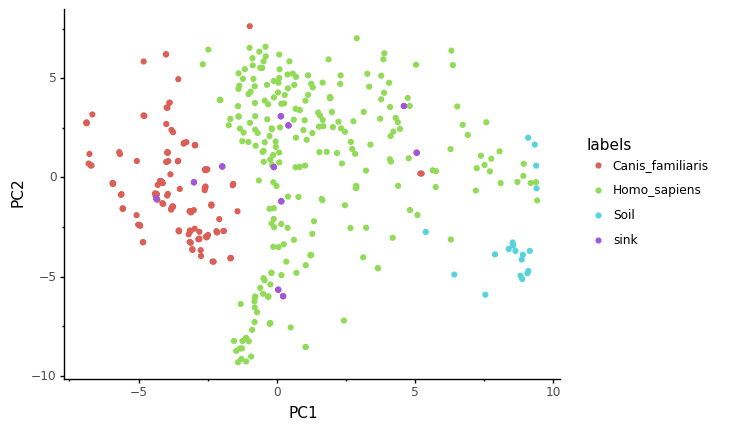:

<ggplot: (297174606)>

:

sp_pred = pd.read_csv("mixed_samples_cnt.sourcepredict.csv", index_col=0)

:

sp_pred.T.head()

:

Canis_familiaris Homo_sapiens Soil unknown
mixed_sample_1 0.262665 0.709352 0.012832 0.015152
mixed_sample_2 0.218900 0.753113 0.012836 0.015152
mixed_sample_3 0.189678 0.769471 0.025776 0.015075
mixed_sample_4 0.255186 0.709368 0.020298 0.015148
mixed_sample_5 0.226581 0.722568 0.035699 0.015152
:

mixed_metadata.head()

:

human_sample dog_sample human_prop dog_prop
mixed_sample_1 SRR7658684 ERR1913675 1.0 0.0
mixed_sample_2 SRR1761664 ERR1915293 0.9 0.1
mixed_sample_3 SRR7658622 ERR1916218 0.8 0.2
mixed_sample_4 SRR059440 ERR1914207 0.7 0.3
mixed_sample_5 SRR7658624 ERR1914667 0.6 0.4
:

sp_res = sp_pred.T.merge(mixed_metadata, left_index=True, right_index=True)

:

from sklearn.metrics import r2_score, mean_squared_error

:

mse_sp = round(mean_squared_error(y_pred=sp_res['Homo_sapiens'], y_true=sp_res['human_prop']),2)
r2_sp = round(r2_score(y_pred=sp_res['Homo_sapiens'], y_true=sp_res['human_prop']),2)

:

p = ggplot(data = sp_res, mapping=aes(x='human_prop',y='Homo_sapiens')) + geom_point()
p += labs(title = f"Homo sapiens proportions predicted by Soucepredict - $MSE = {mse_sp}$ - $R^2 = {r2_sp}$", x='actual', y='predicted')
p += theme_classic()
p += coord_cartesian(xlim=[0,1], ylim=[0,1])
p += geom_abline(intercept=0, slope=1, color = "red", alpha=0.2, linetype = 'dashed')
p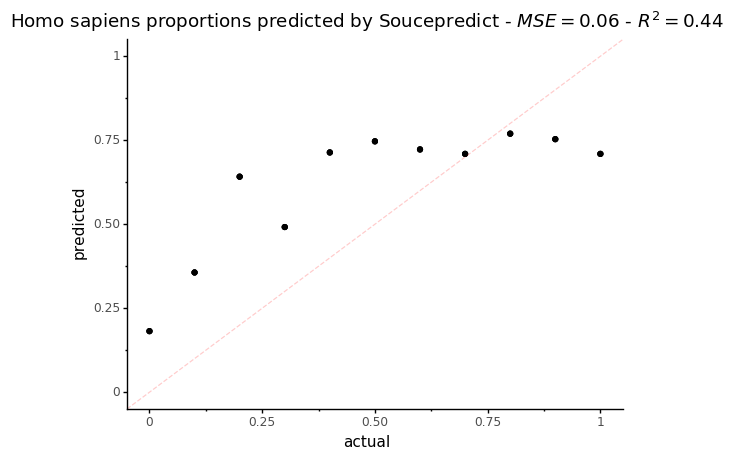:

<ggplot: (-9223372036557649955)>


On this plot, the dotted red line represents what a perfect proportion estimation would give

:

sp_res_hist = (sp_res['human_prop'].append(sp_res['Homo_sapiens']).to_frame(name='Homo_sapiens_prop'))
sp_res_hist['source'] = (['actual']*sp_res.shape+['predicted']*sp_res.shape)

:

p = ggplot(data = sp_res_hist, mapping=aes(x='Homo_sapiens_prop')) + geom_density(aes(fill='source'), alpha=0.3)
p += labs(title = 'Distribution of Homo sapiens predicted proportions by Sourcepredict')
p += scale_fill_discrete(name="Homo sapiens proportion")
p += theme_classic()
p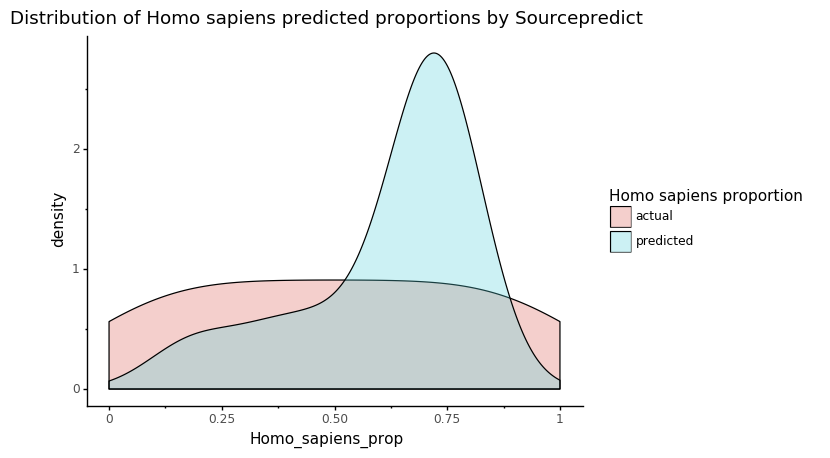:

<ggplot: (-9223372036557649934)>


This plot shows the actual and predicted by Sourcepredict distribution of Human proportions. What we are interested in is the overlap between the two colors: the higher it is, the more the estimated Human proportion is accurate.

## Sourcetracker2¶

Preparing count table

:

cnt_train.merge(mixed_samples, right_index=True, left_index=True).to_csv("st_mixed_count.csv" , sep="\t", index_label="TAXID")

:

!biom convert -i st_mixed_count.csv -o st_mixed_count.biom --table-type="Taxon table" --to-json


:

train_labels['SourceSink'] = ['source']*train_labels.shape

:

mixed_metadata['labels'] = ['-']*mixed_metadata.shape

:

st_labels = train_labels.append(mixed_metadata[['labels', 'SourceSink']])

:

st_labels = st_labels.rename(columns={'labels':'Env'})[['SourceSink','Env']]

:

st_labels.to_csv("st_mixed_labels.csv", sep="\t", index_label='#SampleID')

Running Sourcetracker2 sourcetracker2 gibbs -i st_mixed_count.biom -m st_mixed_labels.csv -o mixed_prop --jobs 6
(Sourcetracker2 was run on a Linux remote server because of issues running it on MacOS)

Sourcetracker2 results

:

st_pred = pd.read_csv("_assets/mixed_prop/mixing_proportions.txt", sep="\t", index_col=0)

:

st_res = st_pred.merge(mixed_metadata, left_index=True, right_index=True)

:

mse_st = round(mean_squared_error(y_pred=st_res['Homo_sapiens'], y_true=st_res['human_prop']),2)
r2_st = round(r2_score(y_pred=st_res['Homo_sapiens'], y_true=st_res['human_prop']),2)

:

p = ggplot(data = st_res, mapping=aes(x='human_prop',y='Homo_sapiens')) + geom_point()
p += labs(title = f"Homo sapiens proportions predicted by Soucepretracker2 - $MSE = {mse_st}$ - $R^2 = {r2_st}$", x='actual', y='predicted')
p += theme_classic()
p += coord_cartesian(xlim=[0,1], ylim=[0,1])
p += geom_abline(intercept=0, slope=1, color = "red", alpha=0.2, linetype = 'dashed')
p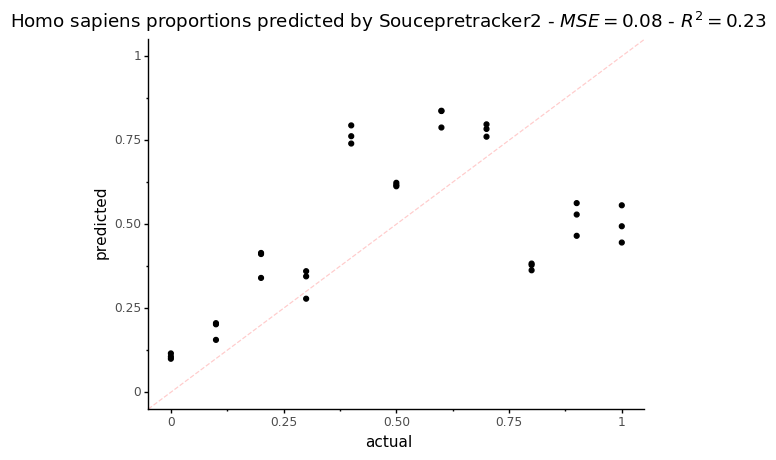:

<ggplot: (297644629)>


On this plot, the dotted red line represents what a perfect proportion estimation would give.

:

st_res_hist = (st_res['human_prop'].append(st_res['Homo_sapiens']).to_frame(name='Homo_sapiens_prop'))
st_res_hist['source'] = (['actual']*st_res.shape+['predicted']*st_res.shape)

:

p = ggplot(data = st_res_hist, mapping=aes(x='Homo_sapiens_prop')) + geom_density(aes(fill='source'), alpha=0.4)
p += labs(title = 'Distribution of Homo sapiens predicted proportions by Sourcetracker2')
p += scale_fill_discrete(name="Homo sapiens proportion")
p += theme_classic()
p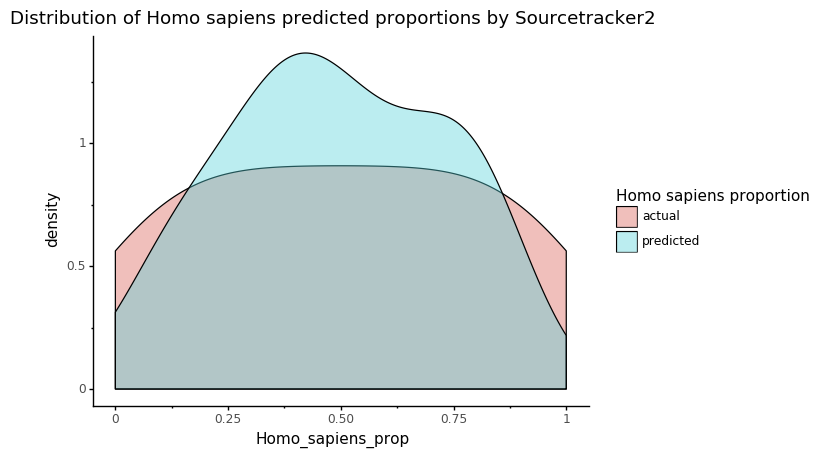:

<ggplot: (297182405)>


This plot shows the actual and predicted by Sourcepredict distribution of Human proportions. What we are interested in is the overlap between the two colors: the higher it is, the more the estimated Human proportion is accurate.

## Conclusion¶

For source proportion estimation in samples of mixed sources, Sourcepredict, especially when using it with -kne all neighbors, performs similarly, or slightly better than Sourcetracker2.
However, Sourcepredict wasn’t designed for source prediction in mind, as opposed to source proportion estimation. Therefore, for source proportion estimation, we still recommend using Sourcetracker2, even if Sourcepredict can perform similarly.